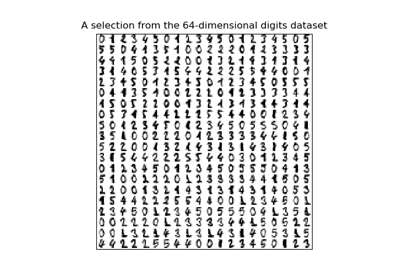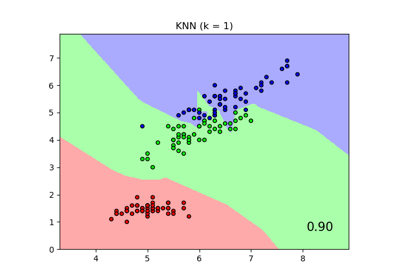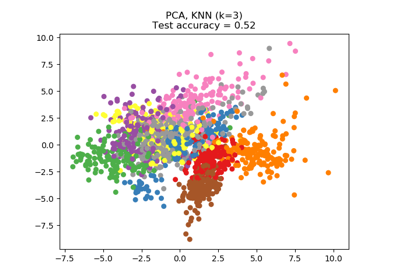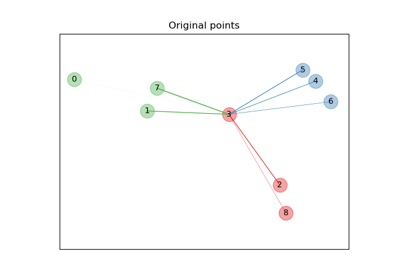# sklearn.neighbors.NeighborhoodComponentsAnalysis¶

class sklearn.neighbors.NeighborhoodComponentsAnalysis(n_components=None, init=’auto’, warm_start=False, max_iter=50, tol=1e-05, callback=None, verbose=0, random_state=None)[source]

Neighborhood Components Analysis

Neighborhood Component Analysis (NCA) is a machine learning algorithm for metric learning. It learns a linear transformation in a supervised fashion to improve the classification accuracy of a stochastic nearest neighbors rule in the transformed space.

Read more in the User Guide.

Parameters: n_components : int, optional (default=None) Preferred dimensionality of the projected space. If None it will be set to n_features. init : string or numpy array, optional (default=’auto’) Initialization of the linear transformation. Possible options are ‘auto’, ‘pca’, ‘lda’, ‘identity’, ‘random’, and a numpy array of shape (n_features_a, n_features_b). ‘auto’ Depending on n_components, the most reasonable initialization will be chosen. If n_components <= n_classes we use ‘lda’, as it uses labels information. If not, but n_components < min(n_features, n_samples), we use ‘pca’, as it projects data in meaningful directions (those of higher variance). Otherwise, we just use ‘identity’. ‘pca’ n_components principal components of the inputs passed to fit will be used to initialize the transformation. (See decomposition.PCA) ‘lda’ min(n_components, n_classes) most discriminative components of the inputs passed to fit will be used to initialize the transformation. (If n_components > n_classes, the rest of the components will be zero.) (See discriminant_analysis.LinearDiscriminantAnalysis) ‘identity’ If n_components is strictly smaller than the dimensionality of the inputs passed to fit, the identity matrix will be truncated to the first n_components rows. ‘random’ The initial transformation will be a random array of shape (n_components, n_features). Each value is sampled from the standard normal distribution. numpy array n_features_b must match the dimensionality of the inputs passed to fit and n_features_a must be less than or equal to that. If n_components is not None, n_features_a must match it. warm_start : bool, optional, (default=False) If True and fit has been called before, the solution of the previous call to fit is used as the initial linear transformation (n_components and init will be ignored). max_iter : int, optional (default=50) Maximum number of iterations in the optimization. tol : float, optional (default=1e-5) Convergence tolerance for the optimization. callback : callable, optional (default=None) If not None, this function is called after every iteration of the optimizer, taking as arguments the current solution (flattened transformation matrix) and the number of iterations. This might be useful in case one wants to examine or store the transformation found after each iteration. verbose : int, optional (default=0) If 0, no progress messages will be printed. If 1, progress messages will be printed to stdout. If > 1, progress messages will be printed and the disp parameter of scipy.optimize.minimize will be set to verbose - 2. random_state : int or numpy.RandomState or None, optional (default=None) A pseudo random number generator object or a seed for it if int. If init='random', random_state is used to initialize the random transformation. If init='pca', random_state is passed as an argument to PCA when initializing the transformation. components_ : array, shape (n_components, n_features) The linear transformation learned during fitting. n_iter_ : int Counts the number of iterations performed by the optimizer.

References

 [Rf9b6baee8229-1] J. Goldberger, G. Hinton, S. Roweis, R. Salakhutdinov. “Neighbourhood Components Analysis”. Advances in Neural Information Processing Systems. 17, 513-520, 2005. http://www.cs.nyu.edu/~roweis/papers/ncanips.pdf
 [Rf9b6baee8229-2] Wikipedia entry on Neighborhood Components Analysis https://en.wikipedia.org/wiki/Neighbourhood_components_analysis

Examples

>>> from sklearn.neighbors.nca import NeighborhoodComponentsAnalysis
>>> from sklearn.neighbors import KNeighborsClassifier
>>> from sklearn.model_selection import train_test_split
>>> X_train, X_test, y_train, y_test = train_test_split(X, y,
... stratify=y, test_size=0.7, random_state=42)
>>> nca = NeighborhoodComponentsAnalysis(random_state=42)
>>> nca.fit(X_train, y_train)
NeighborhoodComponentsAnalysis(...)
>>> knn = KNeighborsClassifier(n_neighbors=3)
>>> knn.fit(X_train, y_train)
KNeighborsClassifier(...)
>>> print(knn.score(X_test, y_test))
0.933333...
>>> knn.fit(nca.transform(X_train), y_train)
KNeighborsClassifier(...)
>>> print(knn.score(nca.transform(X_test), y_test))
0.961904...


Methods

 fit(self, X, y) Fit the model according to the given training data. fit_transform(self, X[, y]) Fit to data, then transform it. get_params(self[, deep]) Get parameters for this estimator. set_params(self, \*\*params) Set the parameters of this estimator. transform(self, X) Applies the learned transformation to the given data.
__init__(self, n_components=None, init=’auto’, warm_start=False, max_iter=50, tol=1e-05, callback=None, verbose=0, random_state=None)[source]
fit(self, X, y)[source]

Fit the model according to the given training data.

Parameters: X : array-like, shape (n_samples, n_features) The training samples. y : array-like, shape (n_samples,) The corresponding training labels. self : object returns a trained NeighborhoodComponentsAnalysis model.
fit_transform(self, X, y=None, **fit_params)[source]

Fit to data, then transform it.

Fits transformer to X and y with optional parameters fit_params and returns a transformed version of X.

Parameters: X : numpy array of shape [n_samples, n_features] Training set. y : numpy array of shape [n_samples] Target values. X_new : numpy array of shape [n_samples, n_features_new] Transformed array.
get_params(self, deep=True)[source]

Get parameters for this estimator.

Parameters: deep : boolean, optional If True, will return the parameters for this estimator and contained subobjects that are estimators. params : mapping of string to any Parameter names mapped to their values.
set_params(self, **params)[source]

Set the parameters of this estimator.

The method works on simple estimators as well as on nested objects (such as pipelines). The latter have parameters of the form <component>__<parameter> so that it’s possible to update each component of a nested object.

Returns: self
transform(self, X)[source]

Applies the learned transformation to the given data.

Parameters: X : array-like, shape (n_samples, n_features) Data samples. X_embedded: array, shape (n_samples, n_components) The data samples transformed. NotFittedError If fit has not been called before.

## Examples using sklearn.neighbors.NeighborhoodComponentsAnalysis¶Manifold learning on handwritten digits: Locally Linear Embedding, Isomap…Comparing Nearest Neighbors with and without Neighborhood Components AnalysisDimensionality Reduction with Neighborhood Components AnalysisNeighborhood Components Analysis Illustration